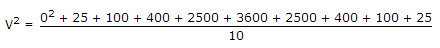# Electronics and Communication Engineering - Electronic Devices and Circuits - Discussion

42.

A periodic voltage has following value for equal time intervals changing suddenly from one value to next... 0, 5, 10, 20, 50, 60, 50, 20, 10, 5, 0, -5, -10 etc. Then rms value of the waveform is

 [A]. 31 V [B]. 32 V [C]. insufficient data [D]. none of these

Answer: Option A

Explanation:RMS value = 965 = 31.064 Volt.

 Dushyant said: (Sep 4, 2014) Why last three value not taken in count?

 Ravi said: (Mar 22, 2016) Because rms value calculated for +ve cycle.

 Rathi said: (Jun 11, 2016) Why there is 10 on the denominator?

 Srivani said: (Jun 13, 2016) @Rathi. Because there are 10 positive cycles on the numerator.

 Balwinder S said: (Aug 17, 2016) I think it should be simply, Vmax / 1.414. Vmax = 60v.

 Manjusihag said: (Sep 1, 2016) Vmax = 60 then Vrms = 0.5, Multiply by 60 = 30, The answer due to +ve half cycle.

 Mohsin said: (Jan 1, 2017) Why? (Vrms =0.5).

 Mukul said: (Apr 4, 2018) Why vm/√2 is not taken here? Please explain.

#### Post your comments here:

Name *:

Email   : (optional)

» Your comments will be displayed only after manual approval.

#### Current Affairs 2021

Interview Questions and Answers